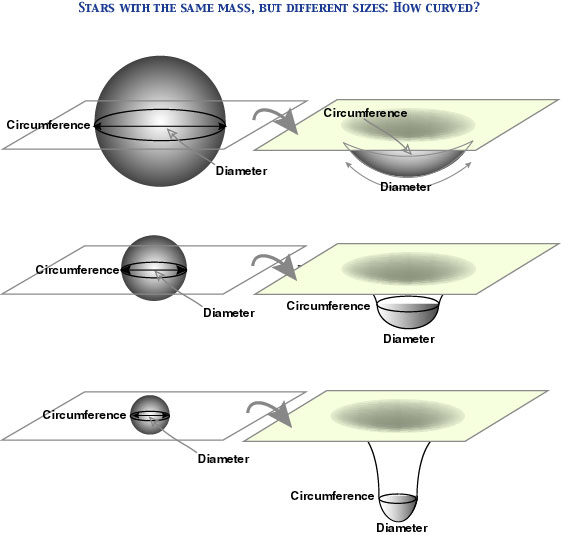# The Field Equations of General Relativity# Keeping It Relatively Simple

The Einstein Field Equations of General Relativity are vast and complex, but they can be written with deceptive simplicity.  Using modern notation, the field equations can be formulated as$\boldsymbol G = \frac {- 8 \pi G}{c^4} \boldsymbol T$

G and T represent complicated mathematical constructs called tensors.  G describes the curvature of space-time, while T describes the distribution of energy and momentum.

Both of these quantities vary with time and position.

The remaining symbols are

• Newton’s gravitational constant  G = 6.673 x 10-11 N m2 kg-2
• the speed of light c = 2.998 x 108 m s-1.

In his 1916 paper, Albert Einstein used these equations of General Relativity to investigate planetary motion in the Solar System, and to predict the non-Newtonian deflection of light by the Sun.

# Einstein’s Big Blunder

The following year, Einstein publishes his first paper on relativistic cosmology, and applies the theory of General Relativity to the space-time geometry of the entire Universe.  While working on that paper, he realises that one of his previous assumptions was unnecessary and inappropriate in the broader context of cosmology.  This “blunder” leads him to introduce an additional term to the field equations$\boldsymbol G + \Lambda \boldsymbol g = \frac {- 8 \pi G}{c^4} \boldsymbol T$

The g is another tensor, while$\Lambda$ is the cosmology constant.

A positive value of$\Lambda$ implies the action of a long-range repulsion that might balance or even overwhelm the attractive influence of gravity.